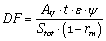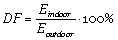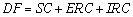# Algorithm / equation daylight factor and rendering engine

I am a student of Building Engineering-Architecture at the University of Pisa and I am preparing my degree thesis in lighting engineering.
I am writing this to you because I am using your software for the analysis of the light factor in order to compare it to the Italian law UNI 10840: 2007 and other similar softwares, such as DIALux evo 8.1 and VELUX Daylight Visualizer 2.
Therefore, I would like to ask you for more information about HoneyBee 0.0.64 and HoneyBee + 0.0.04, in particular about the rendering engine used and the algorithm / equation used to calculate the daylight factor.
Best regards
Luca Bianchi

See the answer to a similar question here:

Also see this for some comparison studies in Italy:

I ask you to confirm the following.

The daylighting software can estimate daylight levels in the room. However, the quality and accuracy of the simulations is influenced by several factors:

1. method of calculation
2. sky model
3. building model
4. properties of the surface
5. user expertise

Let’s suppose now that my calculation is influenced only by the method of calculation and sky model.

HoneyBee Legacy 0.0.64
HoneyBee Legacy uses Daysim software (rendering engine) which is based on Radiance.
In order to realize the rendering Daysim, it uses the Ray tracing technique. Gensky CIE 2002 is used for modelling the sky.

HoneyBee [+] 0.0.04
Since Daysim had some limitations, in HoneyBee [+] what is directly used as a software (rendering engine) is Radiance, which uses the technique of Ray tracing . Gensky CIE 2002 is used for modelling the sky.

VELUX Daylight Visualizer
It is developed by LUXION (https://luxion.com/), same producer of KeyShot.
VELUX Daylight Visualizer uses the Photon mapping technique, but I do not know which one is the rendering engine.
For modelling the sky there are three different models of sky: sunny (12), intermediate (7) and overcast (1). These should be the skies defined by the CIE, but I do not know in which law.

DIALux evo 8.1
DIALux evo 8.1 uses Photon shooting technique (is it the same as photon mapping?), but I do not know which one is the rendering engine.
For modelling the sky there are three different models, described in the CIE 110:1994, such as clear, average and overcast.

These software are compared to the Italian standard UNI 10840: 2007, which gives the following equation to calculate the daylight factor:I would like to understand why the four softwares give me some results which are different from each other and are also different from the Italian regulation UNI 10840: 2007.
Is the difference given by the equation that every software uses to calculate the daylight factor or is it, as I suppose, given by the different techniques (Ray tracing, Photon mapping and Photon shooting) and the different algorithms used for each software? If every software uses different algorithms, the daylight simulation it will also vary.
I think that the softwares, in order to calculate the daylight factor, do not use a simple equation like the Italian law UNI 10840: 2007 does, but they simulate the light and then, for calculating the daylight, they use the simple equation which follows:orBest regards
Luca Bianchi

2 Likes

Honeybee[+] too uses Perez Skies. More info here (the technique in that paper is implemented in HB[+].

You might want to check the results of this new technique specifically for daylighting. I am not sure if this method has been validated yet.

I think that the difference in your results are likely due to the variation in how skies are created in each software. If you get the sky definition right in each case, then your final results should be close (if not identical). It’s somewhat of a challenge to create a custom-defined overcast sky with the Perez Sky Model as it does not allow you to choose between overcast, clear, intermediate etc. Instead the sky luminance distribution is decided based on location, time, direct normal radiation and diffuse horizontal radiation. Conversely, this automatic assignment of skies is pretty useful when we talk of running 8000+ daylight calculations as in the case of annual simulations.

Regards,
Sarith

Now I’m confused!@lb.92 aren’t you looking to compare the calculation for daylight factor? In that case Daysim has nothing to do in the process as well as the Perez Sky. Honeybee uses `Radiance` and `gensky -c -B` to generate the sky. See the batch file that is generated in the study folder when you run a daylight factor simulation.

Yes, my goal is to compare the calculation of the daylight factor.

In the post above, I have listed the five factors that influence the simulation of the software (method of calculation, sky model, building model, properties of the surface, user expertise).
The overcast sky is used to calculate the daylight factor, but it is not guaranteed that all softwares use the same definition.

Then, I have listed the method of calculation and the sky model for each software.

I still don’t understand why the four softwares give me some results which are different from each other and are also different from the Italian regulation UNI 10840: 2007.
Is the difference given by the equation that every software uses to calculate the daylight factor or is it, as I suppose, given by the different techniques (Ray tracing, Photon mapping and Photon shooting) and the different algorithms used for each software? If every software uses different algorithms, the daylight simulation it will also vary.
I think that the softwares, in order to calculate the daylight factor, do not use a simple equation like the Italian law UNI 10840: 2007 does, but they simulate the light and then, for calculating the daylight, they use the simple equation which follows:orBest regards
Luca Bianchi

Both Honeybee and Honeybee[+] use DF = 100 * Eindoor / Eoutdoor using Radiance Raytracing. Here is the break down of the process.

1. Generate a cloudy CIE sky with a certain irradiance using `gensky -c -B`.
2. Calculate illuminance level at sensors using `rtrace` and the sky generated in step 1.
3. Divide the values at each sensor by the value outside which is set in step 1.

Hope it helps.

VELUX Daylight Visualizer uses uses the Dali engine - Luxion light transport algorithms. The software actually runs with 1-16 sky conditions which you can find under “Preferences” or use the shortcut “ctrl+p”. The VELUX Daylight Visualizer sky 16 “Traditional Overcast Sky” is the one that match the Radiance Gensky CIE 2002. It uses a smooth luminance gradiation towards zenith, azimuthal uniformity.

The following article gives in detail information about the ssoftware

ASSESSMENT OF VELUX DAYLIGHT VISUALIZER 2 AGAINST CIE 171:2006 TEST CASES
Article